Next: Modelling The Asymmetry Spectrum of the Vortex State Up: Measuring the penetration depth with TF-SR Previous: The Corrected Asymmetry

# The Relaxation Function

In the vortex state the muons experience a spatially varying field strength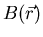. Consequently the x-component of the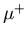polarization may be written: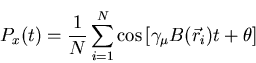(31)

where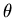is the initial phase. In a real superconductor there are additional contributions to the relaxation rate, so that a more appropriate description of thepolarization is: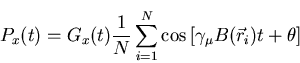(32)

where Gx(t) is a relaxation function (or depolarization function), which describes the damping of the precession signal. The precise form of the relaxation function Gx(t) depends on the origin of the depolarization. In many cases one assumes a gaussian form which is appropriate when the depolarization originates from the muon-nuclear-dipolar interaction .

There is no significant loss of polarization during the short time over which the muons thermalize. This is because the primary interactions by which the muons rapidly lose their initial kinetic energy are electrostatic in nature and hence do not affect the muon spin . Loss of the muon spin polarization in the vortex state is primarily due to the inhomogeneous field distribution, which in turn can be related to the magnetic penetration depth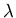. Asdecreases, the spatial variation in the magnetic field becomes greater and there is a corresponding increase in the relaxation rate of the muon spin polarization (see Fig. 3.12). As Fig. 3.1(b) indicates, the field distribution for a perfect vortex lattice is far from being gaussian, but rather is highly asymmetric.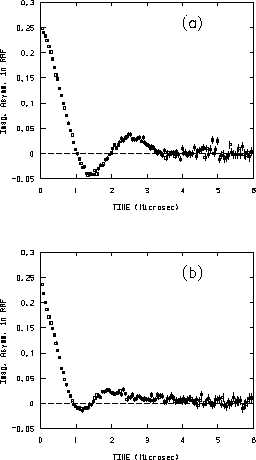The interaction of the-spin with nuclear-dipolar fields in the sample leads to further damping of the precession signal and a corresponding broadening of the field distribution. Normally, a gaussian distribution of the dipolar fields at the-site is assumed. Above Tc, this leads to a Gaussian relaxation function: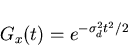(33)

where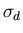is the muon spin depolarization rate due to the nuclear-dipolar fields. The value ofdetermined from data taken above Tc, is assumed to be the same in the superconducting state.

For a real sample in the vortex state, decoration experiments have shown that the vortex lattice is not perfect. Deviations from the ideal flux-line lattice lead to a further relaxation of the precession signal . Consequently we can redefine the relaxation function as: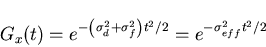(34)

where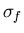is the muon spin depolarization rate due to lattice disorder and any additional depolarizing phenomena and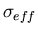is the effective depolarization rate such that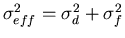. The influence ofis to further broaden the field distribution n(B) beyond that due to.

It should be noted that the additional broadening of the field distribution due to flux-line lattice disorder is difficult to define. Because the field distribution corresponding to an ideal flux-line lattice is highly asymmetric, one would anticipate distortions of the lattice to also be asymmetric in nature. In Eq. (3.34) we are assuming the distortions are gaussian distributed, but it can be shown analytically that convoluting with a gaussian does not change the average field of the distribution.Next: Modelling The Asymmetry Spectrum of the Vortex State Up: Measuring the penetration depth with TF-SR Previous: The Corrected Asymmetry
Jess H. Brewer
2001-09-28